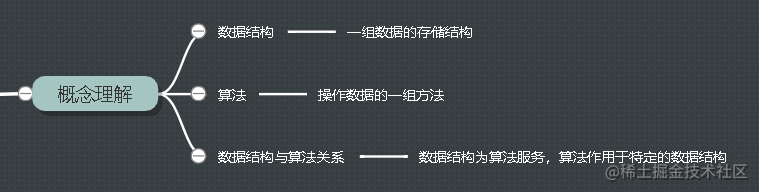时间复杂度和空间复杂度

1. 数据结构与算法关系
2. 时间复杂度
3. 空间复杂度
4. 总结

数据结构与算法关系大O复杂度表示法

int cal(int n) {
int sum = 0;
int i = 1;
for (; i <= n; ++i) {
sum = sum + i;
}
return sum;
}

int cal(int n) {
int sum = 0;
int i = 1;
int j = 1;
for (; i <= n; ++i) {
j = 1;
for (; j <= n; ++j) {
sum = sum +  i * j;
}
}
}

T(n) = O(n)

T(n) = O(2n + 2)
T(n) = O( 2n*n + 2n + 3)

T(n) = O(n)
T(n) = O(n*n)

时间复杂度

O(n)：无论有多少行代码，只有执行次数能确定，那么它的时间复杂度量级就表示为 O(1)，不会出现 O(2)、O(3)等其他情况。

O(logn)：对数时间复杂度计算主要是找到满足的条件，计算代码运行的次数，即代码运行多少次才能执行完，举例如下：

i=1;
while (i <= n)  {
i = i * 2;
}

O(m+n)：如下面这段代码在表示复杂度的时候就不能直接相加去最高阶了：

int cal(int m, int n) {
int sum_1 = 0;
int i = 1;
for (; i < m; ++i) {
sum_1 = sum_1 + i;
}

int sum_2 = 0;
int j = 1;
for (; j < n; ++j) {
sum_2 = sum_2 + j;
}

return sum_1 + sum_2;
}

m 和 n 表示两个数据规模，我们无法确定 m 和 n 谁的量级大，此时的时间复杂度就表示为 O(m) + O(n)，如果 sum_1 * sun_2 则相应的时间复杂度表示为 O(m) * O(n)。

// n 表示数组 array 的长度
int find(int[] array, int n, int x) {
int i = 0;
int pos = -1;
for (; i < n; ++i) {
if (array[i] == x) pos = i;
}
return pos;
}

// n 表示数组 array 的长度
int find(int[] array, int n, int x) {
int i = 0;
int pos = -1;
for (; i < n; ++i) {
if (array[i] == x) {
pos = i;
break;
}
}
return pos;
}

• 最好情况时间复杂分析：指理想情况下执行某段代码的复杂度，对应上述代码就是当在数组中找到满足 if 语句条件的值的时候，上述代码的时间复杂度就是 O(1) 就是最好时间复杂度；
• 最坏情况时间复杂分析：指最糟糕情况下执行某段代码的复杂度，对应上述代码就是永远在数组中找不到满足条件的退出 for 循环，此时上述代码的时间复杂度就是 O(n) 就是最坏时间复杂度；

空间复杂度

void print(int n) {
int i = 0;//栈内存
int[] a = new int[n];//堆内存
for (i; i <n; ++i) {
a[i] = i * i;
}
}

Android